# Very Short Answers - Polynomials Class 9 Notes | EduRev

## Class 9 : Very Short Answers - Polynomials Class 9 Notes | EduRev

The document Very Short Answers - Polynomials Class 9 Notes | EduRev is a part of the Class 9 Course Mathematics (Maths) Class 9.
All you need of Class 9 at this link: Class 9

Question 1. What is p(–2) for the polynomial p(t) = t2 – t + 1?

Solution: p(t) = t– t + 1
⇒ p(–2) = (–2)– (–2) + 1
= 4 + 2 + 1
= 7

Question 2.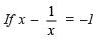then what is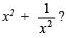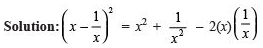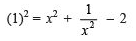⇒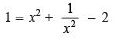⇒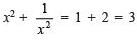Question 3. If x + y = –1, then what is the value of x3 + y3 – 3xy?

Solution: We have x3 + y3 = (x + y)(x2 – xy + y2)
⇒ x3 + y3 = (–1)(x+ 2xy + y2) + 3xy
⇒ x+ y3 = –1(x + y)2 – 3xy
⇒ x+ y3 – 3xy = –1(–1)2 = –1(1)
⇒ x3 + y3 – 3xy = –1

Question 4. Show that p(x) is not a multiple of g(x), when p(x) = x3 + x – 1 g(x) = 3x – 1

Solution: g(x) = 3x – 1 = 0 ⇒ x = 1/3

∴ Remainder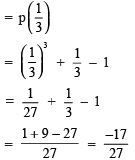Since remainder ≠ 0, so p(x) is not a multiple of g(x).

Question 5. (a) Find the value of ‘a’ if x – a is a factor of x3 – ax2 + 2x + a – 5.
(b) Find the value of ‘a’, if (x – a) is a factor of x– ax2 + 2x + a – 1 [NCERT Exemplar]
(c) If x + 1 is a factor of ax3 + x2 – 2x + 4a – 9 find the value of ‘a’. [NCERT Exemplar]

Solution: (a) Let p(x) = x3 – ax+ 2x + a – 5
since x – a is a factor of p(x),
so p(a) = 0
⇒ (a)– a(a)2 + 2(a) + a – 5 = 0
⇒ (a)3 – a(a)2 + 2(a) + a – 5 = 0
⇒ 3a – 5 = 0 ⇒ a =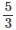(b) Here, p(x) = x3 – ax2 + 2x + a – 1
∵ x – a is a factor of p(x)
∴ p(a) = 0
⇒ a3 – a(a)2 + 2(a) + a – 1 = 0
⇒ a3 – a3 + 2a + a – 1 = 0
⇒ 3a – 1 = 0
⇒ a = 1/3

(c) Here, x + 1 is a factor of p(x) = ax+ x2 – 2x + 4a – 9
∴ p(–1) = 0
⇒ a(–1)3 + (–1)2 – 2(–1) + 4a – 9 = 0
⇒ –a + 1 + 2 + 4a – 9 = 0
⇒ 3a – 6 = 0
⇒ a = 2

Question 6. Without finding the cubes factorise (a – b)3 + (b – c)+ (c – a)3.

Solution: If x + y + z = 0
then x3 + y3 + z= 3xyz
Here, (a – b) + (b – c) + (c –a) = 0
∴ (a – b)+ (b – c)3 + (c – a)3
= 3(a – b)(b – c)(c – a)

Question 7. What is zero of a non-zero constant polynomial?
Solution:
The zero of a non-zero constant polynomial is ‘0’.

Question 8. What is the coefficient of a zero polynomial?
Solution:
The coefficient of a zero polynomial is 1.

Question 9. What is the degree of a biquadratic polynomial?
Solution:
∵ The degree of quadratic polynomial is 2.
∴ The degree of biquadratic polynomial is 4.

Question 10. Is the statement: ‘0’ may be a zero of polynomial, true?
Solution:
Yes, this statement is true.

Question 11. What is the value of (x + a) (x + b)?
Solution:
The value of (x + a) (x + b)
= x2 + (a + b) x + ab.

Question 12. What is the value of (x + y + z)– 2[xy + yz + zx]?
Solution:
∵ (x + y + z)
= x2 + y2 + z2 + 2 xy + 2 yz + 2 zx
= x2 + y+ z2 + 2[xy + yz + zx]
∴ (x + y + z)2 –  2[xy + yz + zx]
= x2 + y2 + z2

Question 13. What is the value of (x + y)3 – 3xy (x + y)?
Solution:
∵ (x + y)3 = x3 + y3 + 3xy (x + y)
∴ [x3 + y3 + 3xy (x + y)] –  [3xy (x + y)] = x3 + y
⇒ (x + y)3 – 3xy(x + y) = x3 + y3
Thus, value of x+ y3 is (x + y)3 – 3xy (x + y)

Question 14. Write the value of x3 – y3.
Solution:
The value of x– y3 is (x – y)3 + 3xy (x – y)

Question 15. Write the degree of the polynomial 4x4 + ox3 + ox5 + 5x + 7?
Solution:
The degree of 4x4 + 0x3 + 0x5 + 5x + 7 is 4.

Question 16. What is the zero of the polynomial p(x) = 2x + 5?
Solution:
∵ p(x) = 0 ⇒ 2x + 5 = 0

⇒ x =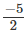∴ zero of 2x + 5 isQuestion 17. Which of the following is one of the zero of the polynomial 2x2 + 7x – 4 ?
2,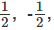, -2 ?

Solution: ∵ 2x2 + 7x – 4 = 2x2 + 8x – x – 4
⇒ 2x (x + 4) – 1 (x + 4) = 0
⇒ (x + 4) (2x – 1) = 0
⇒ x = – 4, x = 1/2,
∴ One of the zero of 2x2 + 7x – 4 is 1/2

Question 18. If a + b + 2 = 0, then what is the value of a3 + b3 + 8.

Solution: ∵ x + y + z = 0
⇒ x+ y3 + z3 = 3xyz
∴ a + b + 2 = 0
⇒ (a)+ (b)3 + (2)3 = 3(a × b × 2) = 6ab
⇒ The value of a3 + b+ 8 is 6ab

Question 19. If 49x2 – p =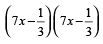, what is the value of p ?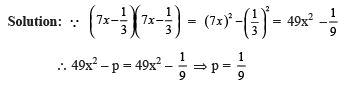Question 20. If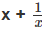= 1, then what is the value of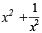?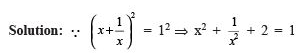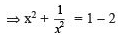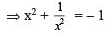Offer running on EduRev: Apply code STAYHOME200 to get INR 200 off on our premium plan EduRev Infinity!

## Mathematics (Maths) Class 9

190 videos|233 docs|82 tests

,

,

,

,

,

,

,

,

,

,

,

,

,

,

,

,

,

,

,

,

,

;